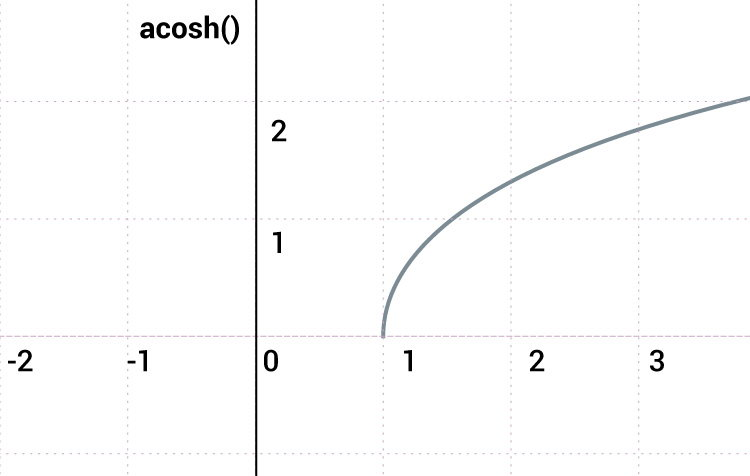# C acosh()

The acosh() function returns the arc hyperbolic cosine (inverse hyperbolic cosine) of a number in radians.The `acosh()` function takes a single argument (x ≥ 1), and returns the arc hyperbolic cosine in radians.

The `acosh()` function is included in `<math.h>` header file.

### acosh() Prototype

`double acosh(double x);`

To find arc hyperbolic cosine of type `int`, `float` or `long double`, you can explicitly convert the type to `double` using cast operator.

``` int x = 0;
double result;
result = acosh(double(x));```

Also, two functions acoshf() and acoshl() were introduced in C99 to work specifically with type `float` and `long double` respectively.

```float acoshf(float x);
long double acoshl(long double x);```

### acosh() Parameter and Return Value

The `acosh()` function takes a single argument greater than or equal to 1.

Parameter Description
double value Required. A double value greater than or equal to 1  (x ≥ 1).

### acosh() Return Value

The `acosh()` functions returns a number greater than or equal to 0 in radians. If the argument passed is less than 1 ( x < 1), the function returns NaN (not a number).

Parameter (x) Return Value
x ≥ 1 a number greater than or equal to 0 (in radians)
x < 1 NaN (not a number)

## Example 1: acosh() function with different parameters

``````#include <stdio.h>
#include <math.h>

int main()
{
// constant PI is defined
const double PI =  3.1415926;
double x, result;

x =  5.9;
result = acosh(x);
printf("acosh(%.2f) = %.2lf in radians\n", x, result);

result = acosh(x)*180/PI;
printf("acosh(%.2f) = %.2lf in degrees\n", x, result);

// parameter not in range
x = 0.5;
result = acosh(x);
printf("acosh(%.2f) = %.2lf", x, result);

return 0;
}``````

Output

```acosh(5.90) = 2.46 in radians
acosh(5.90) = 141.00 in degrees
acosh(0.50) = nan
```

## Example 2: acosh() for INFINITY and DBL_MAX

``````#include <stdio.h>
#include <math.h>
#include <float.h>

int main()
{
double x, result;

// maximum representable finite floating-point number
x =  DBL_MAX;
result = acosh(x);
printf("Maximum value of acosh() in radians = %.3lf\n", result);

// Infinity
x = INFINITY;
result = acosh(x);
printf("When infinity is passed to acosh(), result = %.3lf\n", result);

return 0;
}
``````

Possible Output

```Maximum value of acosh() in radians = 710.476
When infinity is passed to acosh(), result = inf
```

Here, `DBL_MAX` defined in `float.h` header file is the maximum representable finite floating-point number. And, `INFINITY` defined in `math.h` is a constant expression representing positive infinity.

## Example 3: acoshf() and acoshl() function

``````#include <stdio.h>
#include <math.h>
int main()
{
float fx, facosx;
long double lx, ldacosx;

// arc hyperbolic cosine of type float
fx = 5.5054;
facosx = acoshf(fx);

// arc hyperbolic cosine of type long double
lx = 5.50540593;
ldacosx = acoshl(lx);

printf("acoshf(x) = %f in radians\n", facosx);
printf("acoshl(x) = %Lf in radians", ldacosx);

return 0;
}
``````

Output

```acoshf(x) = 2.390524 in radians